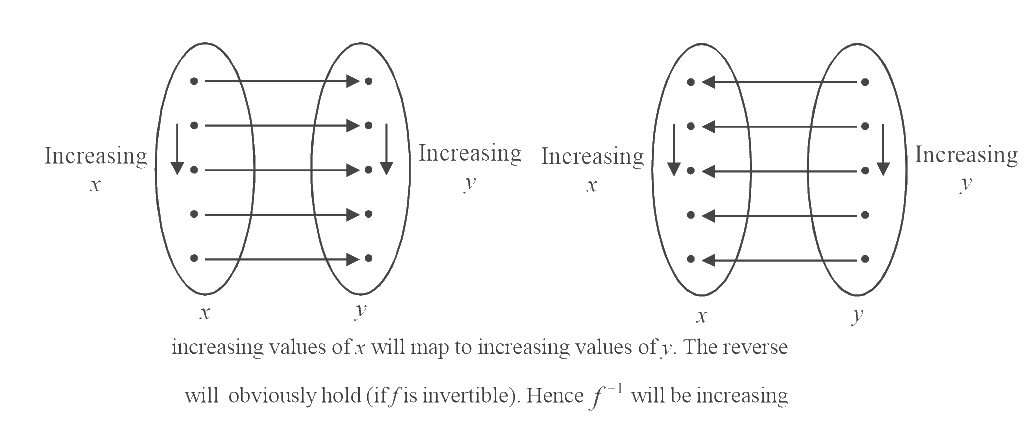# Examples on Inverses of Functions

Go back to  'Functions'

Example - 37

Prove that if $$f$$ is increasing, $${f^{ - 1}}$$ is increasing and if $$f$$ is decreasing, $${f^{ - 1}}$$  is decreasing.

Solution: If $$f$$  is increasing, then $${x_1} < {x_2}$$  implies that  $$f({x_1}) < f({x_2})$$ or $${y_1} < {y_2}$$

Now, just reverse this argument.

For  $${y_1} < {y_2}$$, we should have $${x_1} < {x_2}$$    (from above).

Hence $${y_1} < {y_2}$$   $$\Rightarrow$$ $${x_1} < {x_2}$$, which is equivalent to $${f^{ - 1}}({y_1}) < {f^{ - 1}}({y_2})$$

$$∴ {y_1} < {y_2}$$   $$\Rightarrow$$ $${f^{ - 1}}({y_1}) < {f^{ - 1}}({y_2})$$

$$\Rightarrow$$  $${f^{ - 1}}$$ is increasing.

The decreasing case can be proved similarly

Note: For students who are not convinced, read the following:

(i)  Visualise $$f$$ in the form of a mapping. If $$f$$ is increasing,(ii)   Consider two statements P and Q, where P implies Q, i.e., P $$\Rightarrow$$ Q.

Therefore, not (Q)     $$\Rightarrow$$     not (P)

For example

(Dark clouds are forming in the sky)     $$\Rightarrow$$     (It is about to rain)

(It is not about to rain)     $$\Rightarrow$$     (Dark clouds are not forming in the sky)

Lets apply this to our case. Since f is increasing.

$${x_1} < {x_2}$$   $$\Rightarrow$$    $${y_1} < {y_2}$$   $$\left( {{\rm{where}}\,\,y = f(x),\,\,\,x = {f^{ - 1}}(y)} \right)$$

Hence                    not $$({y_1} < {y_2})$$   $$\Rightarrow$$          not $$({x_1} < {x_2})$$

or      $${y_1} > {y_2}$$       $$\Rightarrow$$      $${x_1} > {x_2}$$

or    $${y_1} > {y_2}$$          $$\Rightarrow$$      $${f^{ - 1}}({y_1}) > {f^{ - 1}}({y_2})$$

Hence, $${f^{ - 1}}$$  is increasing.

Example – 38

If  $$f(x) = \sin x + \cos x$$  and  $$g(x) = {x^2} - 1,$$  then find the interval in which $$g\left( {f(x)} \right)$$  is invertible.

Solution:

\begin{align} g\left( {f(x)} \right) &= g(sinx + cosx) = {(sinx + cosx)^2} - 1\\ &= 1 + 2\sin x\,\cos x - 1 = sin2x \end{align}

We have seen that $$\text{sin}x$$ is invertible in \begin{align}\left[ { - \frac{\pi }{2},\frac{\pi }{2}} \right].\end{align} Therefore, $$\text{sin2}x$$ will be invertible in \begin{align}\left[ { - \frac{\pi }{4},\frac{\pi }{4}}\right].\end{align}

In fact, any function is invertible in all such intervals where it is one-one and onto. $$\text{Sin2}x$$ will be invertible in infinitely many such intervals. But \begin{align}\left[ { - \frac{\pi }{4},\frac{\pi }{4}} \right]\end{align} can be considered the basic invertible domain for $$\text{sin2}x$$ since this interval is centered around the origin.